# Texas Go Math Grade 3 Lesson 4.4 Answer Key Use the Break Apart Strategy to Add

Refer to our Texas Go Math Grade 3 Answer Key Pdf to score good marks in the exams. Test yourself by practicing the problems from Texas Go Math Grade 3 Lesson 4.4 Answer Key Use the Break Apart Strategy to Add.

## Texas Go Math Grade 3 Lesson 4.4 Answer Key Use the Break Apart Strategy to Add

Unlock the Problem

There are more zoos in Germany than in any other country. At one time, there were 355 zoos in the United States and 414 zoos in Germany. How many zoos were there in the United States and Germany altogether?

You can use the break apart strategy to find sums.

Math Talk
Mathematical Processes

Do you think the sum will be greater than or less than 800? Explain
The sum is greater than 800.
355 + 414 = 769
Estimate: 800
Explanation:
The Break Apart Strategy of Addition involves separately adding the ones place, tens place, hundreds place, etc. numbers.
These individual sums are then added together to get the final sum of the original numbers.
Example 1

STEP 1:
Estimate. 400 + 400 = _____

400 + 400 = 800
Explanation:
355 is rounded to nearest hundreds as 400
414 is rounded to its nearest hundreds as 400
So, Estimate. 400 + 400 = 800

STEP 2: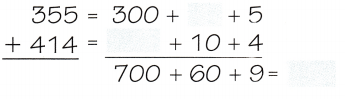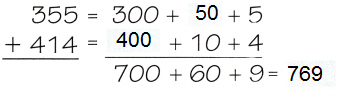Explanation:
The Break Apart Strategy of Addition involves separately adding the ones place, tens place, hundreds place, etc. numbers.
These individual sums are then added together to get the final sum of the original numbers.

STEP 3:
700 + 60 + 9 = _________
So, there were _________ zoos in the United States and Germany altogether.
700 + 60 + 9 = 769
So, there were 769 zoos in the United States and Germany altogether.
Explanation:
The Break Apart Strategy of Addition involves separately adding the ones place, tens place, hundreds place, etc. numbers.
These individual sums are then added together to get the final sum of the original numbers.

Example 2

STEP 1:
Estimate. 300 + 300 = ________
300 + 300 = 600
Explanation:
as 343 is below 350, so 343 is rounded to 300 and
259 is greater then 250, so 259 is rounded to 300 hundred
300 + 300 = 600

STEP 2: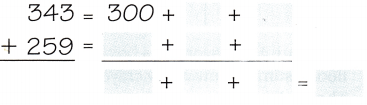Explanation:
The Break Apart Strategy of Addition involves separately adding the ones place, tens place, hundreds place, etc. numbers.
These individual sums are then added together to get the final sum of the original numbers.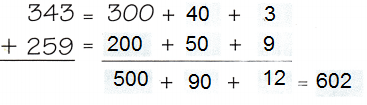STEP 3:
500 + 90 + 12 = __________
So, 343 + 259 = __________
Explanation:
The sum of the addends are same in both the equations.
500 + 90 + 12 = 602
So, 343 + 259 = 602

Math Talk
Mathematical Processes

Explain how the break apart strategy uses expanded forms of numbers.
Explanation:Share and Show

Question 1.
Complete.
Estimate: 400 + 400 = ___________So, 425 + 362 = ___________
Explanation:
The Break Apart Strategy of Addition involves separately adding the ones place, tens place, hundreds place, etc. numbers.
These individual sums are then added together to get the final sum of the original numbers.Estimate. Then use the break apart strategy to find the sum.

Question 2.
Estimate to hundreds. __________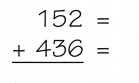Explanation:
Estimate to hundreds.
200 + 400 = 600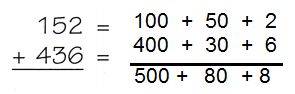Question 3.
Estimate using compatible numbers. ___________Explanation:
Estimate to hundreds.
500 + 200 = 700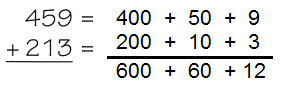Problem Solving

Practice: Copy and Solve Estimate. Then solve.

Question 4.
163 + 205
Explanation:
163 is rounded to 200 and
205 is rounded 200
So, Estimate 200 + 200 = 400

Question 5.
543 + 215
Explanation:
543 is rounded to 500 and
215 is rounded 200
So, Estimate 500 + 200 = 700

Question 6.
213 + 328
Explanation:
213 is rounded to 200 and
328 is rounded to 300
So, Estimate 200 + 300 = 500

Question 7.
152 + 304
200 + 300 = 500
Explanation:
152 is rounded to 200 and
304 is rounded to 300
Estimate 200 + 300 = 500

Question 8.
There are 431 crayons in a box and 204 crayons on the floor. Estimate how many crayons there are altogether. Then solve.
Explanation:
There are 431 crayons in a box and 204 crayons on the floor.
Estimate all crayons together
431 + 204 = 635
431 is rounded to 400 and 204 is rounded to 200
the sum 400 + 200 = 600

Problem Solving

Use the table for 9-10.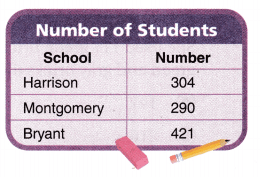Question 9.
H.O.T. Analyze The number of students in Collins School is more than double the number of students in Montgomery School. What is the least number of students that could attend Collins School?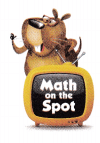Explanation:
Number of students in Montgomery School = 290
The number of students in Collins School is more than double the number of students in Montgomery School i.e.,
290 + 290 =580
Total students in Collin’s School = 580

Question 10.
Multi-Step Which two schools together have fewer than 600 students? Explain.
Both schools have more than 600 students together.
Harrison and Bryant school together  or Montgomery and Bryant
Explanation:
Harrison and Bryant school together
304 + 421 = 725
Montgomery and Bryant school
290 + 421 =  711

Question 11.
H.O.T. What’s the Error? Lexi used the break apart strategy to find 145 + 203. Describe her error. What is the correct sum?Explanation:
203 is written as 230 , is the mistake
it should be written as follows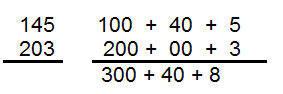Question 12.
H.O.T. Use Math Language Is the sum of 425 and 390 less than or greater than 800? How do you know?
The sum of 425 and 390 is greater than 800
Explanation:
425  = 400 + 20 + 5
390  = 300 + 90 + 0
425 + 390 = 700 + 110 + 5 = 815
425 + 390 =815 is greater then 800

Fill in the bubble for the correct answer choice. Use strategies to solve.

Question 13.
Jen used plastic building bricks to make a Japanese tea house. She used 117 green bricks and 246 gray bricks. How many green and gray bricks did Jen use?
(A) 147
(B) 216
(C) 363
(D) 454
Option (C)
Explanation:
Jen used 117 green bricks and 246 gray bricks.
Total green and gray bricks Jen used
117    =  100 + 10 + 7
246    =  200 + 40 + 6
117 + 246 = 300 + 50 +13 = 300 + 63 = 363

Question 14.
John built two different model log cabins. One used 355 logs and the other used 532 logs. How many logs did John use to make both houses?
(A) 587
(B) 678
(C) 787
(D) 887
Option (D)
Explanation:
One used 355 logs and the other used 532 logs.
Number logs John used to make both
355   =  300 + 50 + 5
532   =  500 + 30 + 2
355 + 532 = 800 + 80 + 7 = 800 + 87 = 887

Question 15.
Multi-Step John is building a tool shed. He has a box of nails that has 300 one-inch nails. He will need all but 13 of the one-inch nails and 312 two-inch nails. How many nails will John use?
(A) 459
(B) 599
(C) 689
(D) 759
Option (B)
Explanation:
John has a box of nails that has 300 one-inch nails.
He will need all but 13 of the one-inch nails and 312 two-inch nails.
Number of nails John used
300 + 13 = 313
312 + 313 = 625
599 is rounded to 600 and 625 is rounded to 600

Texas Test Prep

Question 16.
What is the sum of 421 and 332?
(A) 763
(B) 751
(C) 753
(D) 653
Option (C)
Explanation:
Use break apart strategy
421 =  400 + 20 + 1
332  =  300 + 30 + 2
421 + 332 = 700 + 50 + 3 = 753

### Texas Go Math Grade 3 Lesson 4.4 Homework and Practice Answer Key

Estimate. Then use the break apart strategy to find the sum.

Question 1.
Estimate: ____________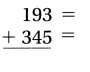Explanation:
193 is more then 150, so 193 is rounded to 200 and
345 is less then 350, so  345 is rounded to 300
200 + 300 = 500

Question 2.
Estimate: ____________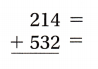Explanation:
214 is less then 250, so 214 is rounded to 200 and
532 is less then 550, so 532 is rounded to 500
200 + 500 = 700

Question 3.
Estimate: __________Explanation: 800
642 is less then 650, so 642 is rounded to 600 and
227 is , less then 250, so  227 is rounded to 200
600 + 200 = 800

Question 4.
Estimate: ____________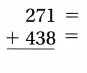Explanation: 700
271 is more then 250, so 271 is rounded to 300 and
438 is , less then 450, so 438 is rounded to 400
300 + 400 = 700

Question 5.
Estimate: ____________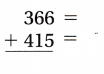Explanation:
366 is more then 350, so 366 is rounded to 400 and
415 is ,less then 450, so 415 is rounded to 400
400 + 400 = 800

Question 6.
Estimate: _____________Explanation:
289 is more then 250, so 289 is rounded to 300 and
274 is ,more then 250, so 274 is rounded to 300
300 + 300 = 600

Problem Solving

Question 7.
How many gallons of white and beige paint were sold?609 Gallons
Explanation:
Number of white gallons = 336
336 = 300 + 30 + 6
Number of Beige gallons = 273
273 = 200 + 70 + 3
Total gallons sold together
336 + 273 = 500 + 100  + 9 = 600 + 9 = 609

Lesson Check

Question 8.
There are two baby giraffes at the zoo. One weighs 148 pounds and the other weighs 137 pounds. Which is their combined weight?
(A) 295 pounds
(B) 275 pounds
(C) 285 pounds
(D) 278 pounds
Option (C)
Explanation:
One baby giraffe weighs 148 pounds
148 = 100 + 40 +8
the other baby weighs 137 pounds.
137 = 100 + 30 + 7
Combined weight for both the babies
148 + 137 = 200 + 70 + 15 = 200 + 85 = 285 pounds

Question 9.
One day, Elmo the elephant ate 128 pounds of food. Ella the elephant ate 115 pounds of food. Together, how many pounds of food did the elephants eat that day?
(A) 244 pounds
(B) 233 pounds
(C) 253 pounds
(D) 243 pounds
Option (D)
Explanation:
Elmo the elephant ate 128 pounds of food.
128 = 100 + 20 + 8
Ella the elephant ate 115 pounds of food.
115 = 100 + 10 + 5
Total pounds of food the elephants ate that day
128 + 115 = 200 + 30 + 13 = 243

Question 10.
There are 176 snakes and 236 birds living at the zoo. How many birds and snakes are there?
(A) 422
(B) 412
(C) 402
(D) 302
Option (B)
Explanation:
There are 176 snakes and 236 birds living at the zoo.
176 = 100 + 70 + 6
236 = 200 + 30 + 6
Birds and snakes together
176 + 236 = 300 + 100 + 12 =412

Question 11.
A zoo rescued 252 animals last year and 328 animals this year. How many animals were rescued in these two years?
(A) 590
(B) 580
(C) 570
(D) 680
Option (B)
Explanation:
A zoo rescued 252 animals last year
252  =    200  + 50   +  2
A zoo rescued 328 animals this year.
328  =    300  +  20  +  8
Total animals rescued in these two years
252+ 328 = 500 + 70 + 10 = 580

Question 12.
Multi-Step Which is the total amount of money donated to the zoo for June, July, and August?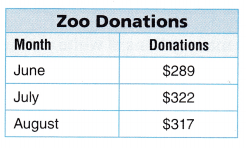(A) $928 (B)$938
(C) $828 (D)$611
Money donated in the month of June = $289 Money donated in the month of July =$322
Money donated in the month of August = $317 The total amount of money donated to the zoo for June, July, and August$289 + $322 +$317 = \$928